Volume 62 Supplement 10

# Prediction of geomagnetic field with data assimilation: a candidate secular variation model for IGRF-11

## Abstract

Data assimilation has been used in meteorology and oceanography to combine dynamical models and observations to predict changes in state variables. Along similar lines of development, we have created a geomagnetic data assimilation system, MoSST-DAS, which includes a numerical geodynamo model, a suite of geomagnetic and paleomagnetic field models dating back to 5000 BCE, and a data assimilation component using a sequential assimilation algorithm. To reduce systematic errors arising from the geodynamo model, a prediction-correction iterative algorithm is applied for more accurate forecasts. This system and the new algorithm are tested with 7-year geomagnetic forecasts. The results are compared independently with CHAOS and IGRF field models, and they agree very well. Utilizing the geomagnetic field models up to 2009, we provide our prediction of 5-year mean secular variation (SV) for the period 2010–2015 up to degree L = 8. Our prediction is submitted to IGRF-11 as a candidate SV model.

## 1. Introduction

There is a long history of geomagnetic measurements on and near the Earth’s surface. Accurate measurements of the geomagnetic field for navigational use have been made for many hundreds of years with reliable records dating back the late 16th century (e.g. Jackson et al., 2000). Measurements at dedicated ground observatories began to be recorded in 1832. Since the early 1960s, satellite measurements have enabled the Earth’s magnetic environment to be monitored from space.

Of the near-Earth measured geomagnetic signals, 97% (in terms of the magnetic energy) are of the internal origin (e.g. Langel and Hinze, 1998). This part of the field, called the core field, is generated and maintained by the geodynamo in the Earth’s fluid outer core. In particular, slow time variations of the core field (the secular variation) are the manifestations of the dynamical processes in the outer core. The remainder arises from various sources, including crustal and oceanic inductions, and electromagnetic processes in the ionosphere and magnetosphere.

These geomagnetic measurements have been used by many research groups around the world to construct global field maps (geomagnetic field modeling). A recent parallel effort is to utilize indirect magnetic measurements, e.g. paleomagnetic and archeomagnetic data for global field modeling. For more details on the global field models, we refer the reader to, e.g. Barraclough (1974), Jackson et al. (2000), Jonkers et al. (2003), Sabaka et al. (2004), Korte and Constable (2005), Korte et al. (2009), Olsen et al. (2009).

The global geomagnetic field can be modeled mathematically by a spherical harmonic expansion. The expansion coefficients, called the Gauss coefficients, can be determined using a least-square inversion from surface and near-surface geomagnetic measurements. The core field can be described by the coefficients up to degree Lobs ≤ 13 (Langel and Estes, 1982; Cain et al., 1989). At higher degrees, it is masked by the crustal magnetic field. Recent studies suggest that it may be possible using current and future satellite measurements (e.g. SWARM mission), to deduce the core field secular variation beyond degree Lobs = 13 (Sabaka and Olsen, 2006; Olsen et al., 2009).

The geodynamo theory can be traced back to Larmor’s (1919) seminal work on the origin of solar magnetism, and is now widely accepted to generate and maintain the core field that varies spatially and temporally. Because of the extremely complex dynamics of rapidly rotating magnetohydrodynamic fluids, analytical dynamo solutions are nearly impossible (except perhaps for unrealistic simple systems). Since the first successful numerical simulation by Glatzmaier and Roberts (1995), we have made substantial progress in modeling the geodynamo. In particular, magnetic fields obtained from various numerical dynamo models with various boundary conditions have displayed properties qualitatively similar to those from surface geomagnetic observations (e.g. Kuang and Bloxham, 1997; Gubbins et al., 2007; Aubert et al., 2008; Sakuraba and Roberts, 2009). For a (not too) recent review on geomagnetism and the geodynamo, we refer the reader to Kono and Roberts (2002).

But these models cannot be directly used to predict geomagnetic secular variations. The numerical magnetic fields have substantial differences with the observations, in particular the non-dipolar components (Kuang et al., 2008, 2009). These differences are due to many factors, including approximations and assumptions used in the models, and numerical parameter mismatches. For example. the numerical Ekman number E (measuring the fluid viscous effect) is many orders of magnitude larger than that appropriate for the Earth’s outer core. This vast parameter gap will be narrowed in time, but unlikely to disappear completely in the next 50 years (estimated based on the past development): over the 12-year period from 1997 to 2009, numerical E is reduced approximately by 1.5 orders of magnitude, from 2 × 10−5 to 5 × 10−7 (e.g. Kuang and Bloxham, 1997; Sakuraba and Roberts, 2009). At the current hardware upgrade rate (doubling computing power every 1.5 years), we would need more than 50 years to reach the Earth-like value E≈5 × 10−14.

Even if the numerical parameter values were appropriate, and the approximations and assumptions were validated, pure numerical simulation will still produce magnetic fields different from those observed, simply because convection in the Earth’s core is very turbulent, and thus the time variation of the core state is very chaotic. This implies that small initial differences in numerical solutions will grow rapidly in time. This situation is reminiscent of modeling atmospheric and oceanic circulations.

However, as we have learned from atmospheric and oceanic studies, assimilation of data and dynamical models can bring numerical solutions closer to the real geophysical state. Results of observing system simulation experiments (OSSEs) on simple magnetohydrodynamic system (Sun et al., 2007) and on full dynamo models (Liu et al., 2007) have shown that by assimilating magnetic data into the model output, the predicted solution can be drawn closer to the true state in a dynamically consistent manner. Using the geomagnetic field model output and the geomagnetic data assimilation system MoSST-DAS, Kuang et al. (2008, 2009) also found that the predicted field is similar to the observations. Similar results are obtained with assimilation based on simpler systems with, e.g., a two-dimensional, quasi-geostrophic flow (Canet et al., 2009), or a steady flow (Beggan and Whaler, 2009).

Among others, these results suggest two significant geophysical applications: using observations to constrain numerical geodynamo models, and using the improved model output to predict geomagnetic secular variation (SV).

This paper focuses on the second application, SV prediction, with an explicit goal to provide an SV candidate model to the IGRF system. This application demands not only a theoretical understanding of the numerical dynamo model and geomagnetic data, but also practical techniques to improve the prediction accuracy. Both require proper assessment of model and observational errors.

In our past work, we have assumed that errors from observations (actually the errors from geomagnetic field models) are negligible compared to dynamo model errors (Kuang et al., 2008, 2009). This is reasonable for assessing the impact of observations on dynamo model solutions, but is expected to be insufficient for providing SV forecast to IGRF.

For this purpose, we introduce in our geomagnetic data assimilation system, MoSST-DAS, a “boot-strap” type of prediction-correction analysis algorithm to reduce forecast errors, and we also use time series of geomagnetic field models to estimate observational errors. This approach is benchmarked with independent field models. And it is the basis for our SV candidate model to IGRF for the period from 2010–2015.

This paper is organized as follows: mathematical formulation of our geomagnetic data assimilation system is summarized in Section 2. Testing of the forecast with observations is described in Section 3. The details of the SV forecast for the period 2010–2015 are provided in Section 4. Discussion is given in Section 5.

## 2. Geomagnetic Data Assimilation System

The geodynamo is described by a set of nonlinear, magnetohydrodynamic equations. In the reference frame co-rotating with the mean angular velocityΩof the solid mantle, they are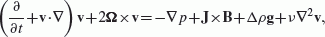(1)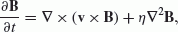(2)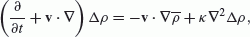(3)

where v is the velocity field, B is the magnetic field, J × B/μ(μis the magnetic permeability) is the current density,Δρis the relative density anomaly (from temperature variation, compositional variation, or both),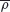is the background conductive state (scaled by the mean density ρ0), v is the kinematic viscosity, η is the magnetic diffusivity, and κ is the dissipation for density anomaly. In the numerical dynamo model used here (Kuang and Bloxham, 1999; Kuang and Chao, 2003; Jiang and Kuang, 2008), this set of equations are nondimensionalized, and are solved via a hybrid finite difference/pseudo-spectral algorithm. After discretization, they can be described by a simple form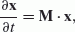(4)

where x is the dynamo state vector; M is the model operator, a nonlinear function of x.

There are a number of different assimilation algorithms developed in the past, most of them have been associated with weather forecasting (Talagrand, 1997). We have employed a sequential assimilation algorithm in our geomagnetic data assimilation system, MoSST-DAS (Kuang et al., 2008). This approach can be summarized as follows. Denote by y the observation: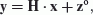(5)

where H is the projection operator, and z° is the observation error. For geomagnetic observations, y includes only the Gauss coefficients of the poloidal field at the Earth’s surface for up to degree L ≤ 13. This is only a small fraction of the generated magnetic field in the core: the magnetic field B in the outer core can be decomposed into the toroidal BT and the poloidal B P fields: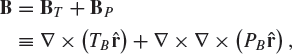(6)

where T B and P B are the toroidal and poloidal scalers, respectively. These scalars can be described by spherical harmonic expansions. For example, in our numerical dynamo modeling,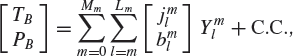(7)

where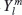are the fully normalized spherical harmonic functions of degree l and order m, and C.C. stands for the complex conjugate. The spectral coefficients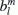of degree l and order m at the top of the D″-layer rd have unique one-to-one relationships with the Gauss coefficients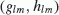used in geomagnetic field modeling: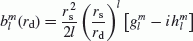(8)

where r s is the mean radius of the Earth’s surface, and i is the imaginary unit number (additional factor will be added to the formulation if different normalization rules are used in field modeling and geodynamo modeling). Therefore we use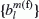for the model forecasts, and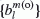for the observations (field model coefficients). The latter can be derived via (8) from the Gauss coefficients provided by the geomagnetic field models.

In numerical dynamo simulation, the truncation orders L m Lobs. It should be pointed out that the spectral coefficients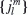in (7) are for the toroidal magnetic field, and are therefore not observable at the surface. In addition to these, the velocity field and the density are also among the unobservable state variables.

In the dynamo model, the velocity field v is described similarly as (6) and (7), and the density anomaly Δρ is also approximated with a truncated spherical harmonic expansion. All spherical harmonic coefficients are defined at discrete radial grid points (e.g. Kuang and Bloxham, 1999). Therefore, the dynamo state, i.e. the numerical model output, at any time can be described by an array x of all spectral coefficients on all radial grid points.

At a sequence of times t a (which are often called the analysis times), the model output xf, (the forecast), is modified through the analysis equation,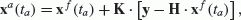(9)

where K is the gain matrix, and can be determine via, e.g., minimizing the error between the analysis xa. xa and the true state xt. The analysis xa. xa will be used as the initial condition for model simulation starting from t a . This process repeats at each analysis time. The difference between the two adjacent analysis time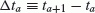(10)

is called the analysis period in this paper. We refer the reader to Kuang et al. (2008, 2009) for the details of the algorithm.

In MoSST_DAS, the scaled coefficients are used in obtaining the analysis xa. xa (Kuang et al., 2009):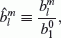(11)

(the ratio of the multiple components to the axial dipole field). Mathematically, this is equivalent to using only field declination and inclination in assimilation. The field intensity is not considered. Because of this, we also call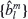the directional coefficients in this paper.

Accordingly, we also use the (non-dimensional) scaled forecast field (with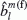) and the scaled observation (with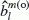) in our analysis. In addition, the mean square field and error are defined as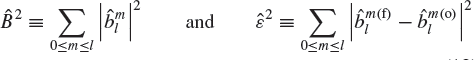(12)

The full forecast field and observations will then require utilization of the axial dipole component. We will discuss this further in the following Sections 4 and 5.

## 3. Prediction-Correction Iterative Algorithm

The model output xf (t) at any time t in an analysis period t a < t < ta+1 is the forecast based on the observations made up to time t a . The difference between the forecast xf and the state x from a pure dynamo simulation (4) describes the correction due to the assimilation of the observations on the dynamo model solutions.

The forecast error (or the forecast accuracy) can be measured by the difference between xf and the true state xt. In our study, xt is the true core state and is unknown (true in nearly all data assimilation applications). Therefore, forecast accuracies are often examined by comparing the observable component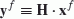(13)

with data. To simplify the discussion, we assume that observations are perfect, i.e. the errors in data are negligible.

If we could make perfect forecasts, then yf(t) = y(t). However, as shown in Fig. 1, they are different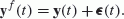(14)

This difference, the forecast error, arises from the model errors (in the perfect observation approximation). By (9), it vanishes at the analysis time ε(t a ) = 0. As shown in the figure, the forecasts gradually depart further away from the observations over longer forecast length, i.e. ε increases with t.

Could this error be reduced for improved predictions? To find an answer, we need to understand first the properties of the errors.

The departure of the forecasts is the result of both model errors and imperfect initial conditions. Therefore, in addition to the true changes in the observations, the increment at a later time t a + δt after the analysis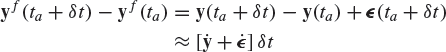(15)

includes also the error growth.

The error ε will be reduced if there are sufficient data to bring the dynamo model solutions closer to the true state in the outer core. But it can not vanish completely since the model is not perfect. However, for the purpose of forecasting, it could be reduced via a “bootstrap” approach, provided that the time scales of the error growth is different from the forecast length.

Mathematically, this could be done by comparing multiple time-varying sequences. To explain the methodology, we consider here two sequences of assimilation starting from two different, but very close analysis times t a and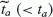. By (14), the forecasts of the two sequences at a time increment δt from the analysis time areThe difference of the two sequences of forecasts is therefore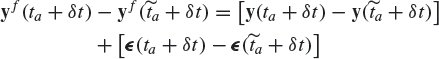(16)

The first term on the right hand side is the true variation of the observations. The second term, the model error, can be approximated via Taylor series expansion: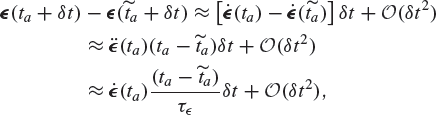(17)

where τ ε is the typical time scale of the error ε. Comparing with (15), we observe that the model error in the estimation (16) is much smaller if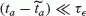.

The model error time scaleτ ε depends on both the model and on the duration of the sequential assimilation. Therefore it can only be assessed via actual experiments. We also call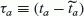(18)

the reanalysis time interval. In general, it should be much smaller than the analysis period Δt a in (10).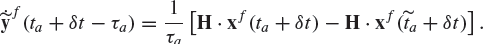(19)

And the corrected geomagnetic forecast yf will then be calculated via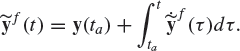(20)

With this procedure, we expect that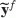will be more accurate than the original uncorrected forecasts (Hxf ), and are therefore often called the “corrected forecasts”.

## 4. SV Prediction: Experiment and Assessment

To test the applicability of the prediction-correction approach (19) and (20), we use the following procedure: first, prior to the application of (19), an assimilation run is made with the longest available observational record, i.e. the Gauss coefficients from paleomagnetic and archeomagnetic data (Korte and Constable, 2005), historical magnetic data (Jackson et al., 2000), ground observatory and satellite data (Sabaka et al., 2004). Combined, they provide up to 7000 years of observations.

In our experiments, the maximum degree Lobs of the Gauss coefficients used in the analysis (9) changes from Lobs = 6 before 1620 (the paleomagnetic field model output), and Lobs = 8 from 1620 to the present day (historical, observatory and satellite records). Thus we have used the maximum number of observations in order to achieve our best estimates of the core state.

Next, we carry out the prediction-correction approach (19) and (20) for the past decade, because continuous satellite measurement during this period provides by far the best global description of the core field, in particular its SV. In addition, two sets of field models are used for the validation analysis: one is CM4 (Sabaka et al., 2004) used to produce sequences of assimilation results; and the other is CHAOS-2s model (Olsen et al., 2009) used for comparing the forecast with observations.

The CM4 is a field model of the quiet-time, near-Earth magnetic field from 1960 to 2002.5. It is derived with a comprehensive approach, using POGO, Magsat, ørsted and CHAMP satellite data. In this approach, all magnetic sources are co-estimated, resulting in optimal separation of the fields (Sabaka and Olsen, 2006). CM4 uses the minimum mean square estimator with the assumptions that the estimator is a linear function of the data, and that the errors are isotropically and independently distributed. This approach is the so-called best, linear, unbiased estimator (BLUE) (Sorenson, 1980). In this model, the core and lithospheric fields are described by a spherical harmonic expansion up to degree and order 65. The SV part is represented by cubic B-splines (with a 2.5-year knot spacing) through degree and order 13.

The CHAOS-2s model is a state-of-the-art model of the geomagnetic field for the last decade, constructed with data from the three satellite missions CHAMP, ørsted and SAC-C, with the internal field secular variation parameterized with order 5 B-splines, lithospheric field to degree 60 and a model of the large-scale magnetospheric field (plus Earth-induced counterpart) allowing for components modeled both in a fixed-Earth and a fixed-Sun reference frame.

In our assimilation, the Gauss coefficients from the both field models are treated as indistinguishable from the “true field” at any particular epoch away from the edges of the models (i.e. the errors in the field models are negligibly small), consistent with the assumption made in the analysis (9) that the errors from current numerical geodynamo models are assumed substantially larger than those from the field models (Kuang et al., 2008, 2009).

We have carried out two forecast experiments: the first covers the period from 1994 to 2001, and the second from 2000 to 2007. The first is to demonstrate the improvement of the forecasts, while the second is used to compare the accuracies of the forecasts with previous IGRF effort (e.g. Mandea and Macmillan, 2000; Macmillan and Maus, 2005).

In both experiments, a 7-year forecast from MoSST_DAS is compared with the observations of the same period to assess the improvement of the prediction-correction approach (19) and (20). The 7-year forecast period chosen in the experiments directly aims at producing IGRF SV candidate models. The IGRF provides forecast only for the 5-year period after the given epoch (e.g. for the period from 2010 to 2015 for IGRF-11 defined in the year 2010). But in practice, the actual analysis could only utilize observations at most up to 0.5 year prior to the epoch. In addition, the B-splines used in geomagnetic field modeling generate end-point effects, which are expected to affect the coefficients at least in the last knot interval of the splines (private communication with Sabaka and Olsen). From these considerations, a 7-year forecast experiment would suffice for the IGRF candidate model objective. But, in terms of general geomagnetic data assimilation, we can provide forecasts for much longer periods (Kuang et al., 2008, 2009). Of course the forecast errors increase with time (eventually reaching the values defined by the differences between the unconstrained dynamo simulation results and the observations).

Before the experiments, we need to determine the time scale τ ε of the model error ε variation in the assimilation, which will then be used to select the reanalysis time interval ta. For this purpose, 4 sequences of 5-year forecasts are carried out: the first starting from 1993; and each following sequence starting one year later than the previous sequence. This implies that the reanalysis time interval is one year if they are used for the prediction-correction process.

In Fig. 2 are the mean square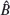of the 4 sequences and of the observations. From the figure we can not find any visible difference among the trends of these sequences, indicating that the forecast error growths at different analysis time are very similar.

To quantify the error growth, we list in Table 1 the scaled error ê defined in (11) at the top of the D″-layer for the 4 sequences. The errors of each forecast sequence are in one row, and the values are provided from the first year to the last (fifth) year of the forecast period. As shown in the table, the errors of the same forecast length (e.g. third-year forecast) are nearly the same: the relative differences among the 5-year forecast errors (in the last column) are less than 1%. These results show that, from 1993 to 1996, the model system error remains approximately unchanged, i.e. its time scaleτ ε (which is not precisely determined) is much longer than 1 year. Therefore, we choose the reanalysis time interval τ a = 1 year in our two experiments.Table 1. The scaled forecast errors at the top of the D″-layer of the 5-year forecasts from the analysis time t a = 1993, 1994, 1995 and 1996, respectively. The errors from a given analysis time are in the same row, and are listed from the year 1 to year 5 of the forecast period.

The first 7-year forecast experiment is from 1994 to 2001. In this experiment, we first make two sequences of forecasts: one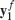starting from 1993, and the other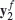starting from 1994, i.e.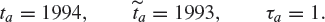By (19), the corrected annual averaged SV forecast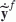in each year t starting from 1994 is then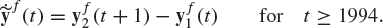(21)

The corrected field forecast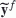from 1994 will be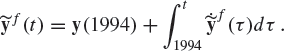(22)

The results are shown in Fig. 3. From the figure we can observe clearly that the corrected forecastsare more accurate than the original uncorrected forecast yf: the maximum difference in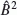is reduced from 0.0015 for yf to 0.00025 for. This is equivalent to approximately 40 nT misfit in the actual field at the top of D″-layer, provided that the dipole coefficient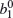is accurately predicted. But, as we shall find in the next section, the actual misfit will be larger, sincecannot be accurately predicted.

The above experiment is not complete: the assessment is not made independently since all utilized observational information is provided by a single source (CM4). Therefore, we carry out the second experiment: we produce a 7-year forecast for the period from 2000 to 2007 based on CM4 field coefficients up to year 2000 (CM4 provides the coefficients up to 2002.5). The process here is similar to that in the first experiment, but with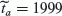and t a = 2000. The corrected field and SV forecasts are then compared with those from CHAOS-2s, IGRF-8 and IGRF-9.

Selection of both IGRF-8 and IGRF-9 in this second experiment is intended for examining the lower and upper bounds of the MoSST_DAS forecast accuracies. IGRF-8 was determined by a task Force at the end of 1999 so that as many ørsted data could be incorporated as possible (Lowes, 2000; Olsen et al, 2000). In terms of data utilization, IGRF-8 SV model for 2000–2005 is the true 5-year forecast, thus the closest to our forecast experiment setting. On the other hand, IGRF-9 was the revision of IGRF-8 in 2003, and was produced by the participating members of IAGA working group V-8 (IAGA Working Group report, 2003). In this revision, observations up to later 2002 were utilized to produce SV from 2000 to 2005. From this point of view, IGRF-9 produced actually a 2.5-year forecast. We expect therefore that its SV forecast is more accurate than that of IGRF-8, and perhaps more accurate than our 7-year SV forecast. The latter implies that IGRF-9 can be a good test case for the upper bound of MoSST_DAS SV forecast accuracy.

Before making the comparison, we need to convert our results to IGRF format. As we stated earlier, MoSST_DAS provides the forecast on the field directional distribution (11). Therefore, the variation of the coefficients from t a to t a + δt is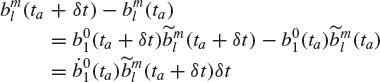(23)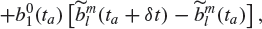(24)

where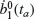is the SV of the axial dipole at the analysis time t a . Both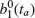andare known when we make field predictions. In the following discussions, we call (23) the “updated assimilation forecast” (UAF) in which the axial dipole field at the later time t a + δt is updated via linear extrapolation of the time series up to t a . We also name (24) the “constant assimilation forecast” (CAF).

Field forecast comparisons are made at the epoch 2005 (i.e. δt = 5 years). In this case, the 2005 field and SV coefficients from CHAOS-2s are treated as the “observation”, and are used to estimate the accuracies of the four different predictions: IGRF-8, IGRF-9, MoSST_DAS UAF and MoSST_DAS CAF. The results are shown in Fig. 4.

In the figure, the solid line is the CHAOS-2s (observation) field model spectrum. The remaining curves are the rms differences between the observation and the forecasts of IGRF-8 (dotted line with upward triangles), IGRF-9 (dotted line with stars), MoSST-DAS CAF (dashed line with circles), and MoSST-DAS UAF (dashed line with diamonds). As shown in the figure, CAF and UAF are nearly identical. This is not surprising, since the forecasted field is derived from the SV forecast via (20). We can also observe from the figure that, as expected, the MoSST-DAS field forecasts are more accurate than IGRF-8, but less accurate than IGRF-9. This simply reflects the fact that IGRF-9 actually provides a shorter period forecast with more data.

The SV forecast results are shown in Fig. 5. The observed SV from 2000 to 2009 are calculated from CHAOS-2s field model. But plotted in the figure are only the spectra of the observed 5-year mean SV from 2000 to 2005 (solid line with squares) and 7-year mean SV from 2000 to 2007 (solid line with asteroids). Since IGRF-9 includes data up to early 2003, we simply assume its 5-year SV forecast could be extended to the 2004–2009 period for accuracy comparison. In addition, IGRF-10 5-year SV forecast is used for the period from 2005 to 2009. In Fig. 5, the rms differences between the CHAOS-2s SV and the MoSST_DAS CAF SV forecasts are smaller than all IGRF SV forecasts. In particular, the difference for the MoSST_DAS 7-year SV forecast is smaller than those of IGRF-9 and IGRF-10. Again, as expected, IGRF-8 SV forecast is the worst among all. Though the improvement is small, but it shows that MoSST_DAS forecasts are at least comparable to the IGRF models.

These independent assessments, in particular with respect to previous IGRF models, provide us confidence in our ability to accurately predict geomagnetic field, in particular SV, with our geomagnetic data assimilation system MoSST_DAS.

## 5. Predicted SV Model for IGRF

IGRF provides predictive averaged SV over a 5-year period starting from a given epoch. Specifically, IGRF-11 will provide the averaged SV for the period from 2010 to 2015. Therefore (19) will be used for our candidate model to IGRF.

In this part of the forecast, CHAOS-2s is used to provide observations from 2002 to 2010. A mathematical smooth transition is used to migrate the Gauss coefficients of CM4 to those of CHAOS-2s in the time period from 2000 to 2002. Since both models agree very well in this overlapping period, the transition is introduced only as a precaution.

The complex spherical harmonic coefficients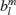defined in (7), are used in the numerical dynamo simulation. They can be converted to the real Gauss coefficients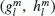used in traditional geomagnetic field representation via (8):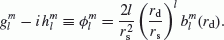(25)

Therefore the forecasts (23) and (24) can be modified accordingly for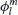. For example, the CAF (24) can be rewritten as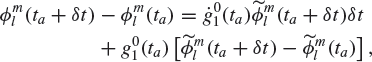(26)

where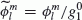are the scaled coefficients of degree l and order m, and t a = 2010. If we define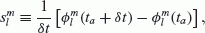(27)

then (26) can be simplified as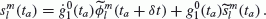(28)

The axial dipole field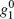and its SV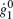in 2010 are determined from CHAOS-2s field coefficients, while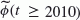and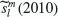are given by MoSST_DAS. The approach is identical to that used in our benchmarking example (discussed in the previous section):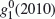is extrapolated via a standard polynomial fit (the difference between the extrapolated results from the second order and the fourth order polynomials is negligible). The SV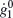of the axial dipole field in 2010 is set to be the mean of the 5-year average SV of CHAOS-2s from 2001 to 2009.

To estimate the forecast misfit, we need to know the uncertainties of all 4 quantities in (28):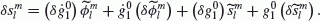(29)

The uncertainties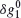and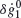of the axial dipole field are given by the standard deviations of the extrapolation and the averages. Thus, the final axial field and the uncertainties are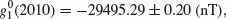(30)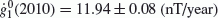(31)

To estimate the uncertainties of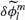(and thus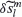), we use the averaged errors of our forecast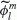for the period from 2004 to 2009 (again compared to CHAOS-2s field in the same period), and the results are shown in Fig. 6.

Our final 5-year forecast for the 2010–2015 period is listed in table 2. Its spectrum is shown in Fig. 9.

## 6. Discussion

Advances in numerical geodynamo modeling over the past 15 years, and the long history of global geomagnetic field observations have made geomagnetic data assimilation possible. Assimilation of the geomagnetic observations with numerical geodynamo models will help validate and improve the approximations and assumptions made in the models, thus help to better understand the dynamo solutions and the dynamical states in the Earth’s outer core. The improved numerical geodynamo models, on the other hand, will help us better interpret geomagnetic observations, and predict geomagnetic secular variations. This paper provides the first attempt at predicting SV using our geomagnetic data assimilation system MoSST_DAS.

In MoSST_DAS, over 7000 years of the geomag-netic/historical/paleomagnetic records are assimilated into the core dynamics model (MoSST) via a sequential assimilation algorithm (9): at each analysis time t a , the model forecasts are modified with the data and are then used as the initial states for future forecasts.

To improve the forecast accuracy, we introduce a “bootstrap” type prediction-correction technique (19)(20) to reduce the model error. The idea behind this technique is very simple: we utilize two sequences of the forecast with slightly different analysis time t a and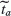. The difference between the two sequences will then remove much of the model errors, i.e. ε in (14), varying on time scales much longer than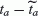. This difference will then be used to improve the secular variation (19), and then the field forecasts (20).

This technique has been tested in two cases. In the first case, observations prior to year 1994 are used for the forecast for the period from 1994 to 2002. As shown in Fig. 3, the technique reduces the forecast error ε by nearly an order of magnitude. In the second case, the last analysis time is set at t a = 2000. And the forecast is made for the following 7 years. The forecast is then compared with CHAOS-2s, IGRF-8 and IGRF-9. As shown in Figs. 4 and 5, the forecasted field and SV from MoSST_DAS agree very well with the observations (CHAOS_2s field model). In addition, the MoSST_DAS field forecast accuracies are at least comparable to IGRF forecast (better than IGRF-8 but slightly worse than IGRF-9 which utilizes more observations for shorter period forecast). However, among all SV forecasts, those from MoSST-DAS are the closest to the observations (Fig. 5).

Using this technique, we produce a MoSST_DAS candidate model for IGRF-11: a 5-year geomagnetic secular variation model for the period from 2010 to 2015, as shown in Fig. 9 and table 2.

This is the first ever attempt of using geomagnetic data assimilation to forecast SV. In this approach, the dynamics of the Earth’s core that we know so far are utilized to make predictions of future changes in the core field. This is very different from techniques used in the past IGRF SV forecasts. Obviously there are many improvements that can be made to this approach.

In addition to improvements to numerical dynamo models and assimilation algorithms (which depend on further understanding of the assimilation solutions, model responses to observational constraints, dynamo properties, etc), we should also consider more direct integration of geomagnetic data into MoSST_DAS. Currently, we use the Gauss coefficients from various field models as the “real observations”. But these coefficients are derived products. Therefore, in the MoSST_DAS, we also inherit all improvements as well as limitations of these field models. One concern is the assessment of the observational errors. They are neglected under the assumption (still largely true) that the model errors are far greater. However, a better forecast needs inclusion of the data errors as well. But it is difficult to assess these errors in the field model Gauss coefficients. For example, any discrepancy between two field models (e.g. CM4 and CHAOS-2s) in an overlapping observation period, different types of data used in the field models, end-point effects (private communication with Jackson, Finlay, Olsen and Sabaka) will bring further complications on this issue. Direct assimilation of the data might help avoid some of these complications. But, any effort will be evaluated by the forecast accuracies.

## References

• Aubert, J., H. Amit, G. Hulot, and P. Olson, Thermochemical flows couple the Earth’s inner core growth to mantle heterogeneity, Nature, 454, doi:10.1038/nature07109, 2008.

• Barraclough, D. R., Spherical harmonic analysis of the geomagnetic field for eight epochs between 1600 and 1910, Geophys. J. R. Astron. Soc., 36, 497–513, 1974.

• Beggan, C. D. and K. A. Whaler, Forecasting change of the magnetic field using core surface flows and ensemble Kalman Filtering, Geophys. Res. Lett., 36, doi:10.1029/2009GL0399, 2009.

• Cain, J., Z. Wang, D. R. Schmitz, and J. Meyer, The geomagnetic spectrum fro 1980 and core-crustal separation, Geophys. J. Int., 97, 443–447, 1989.

• Canet, E., A. Fournier, and D. Jault, Formal and adjoint quasi-geostrophic models of the geomagnetic secular variation, J. Geophys. Res., 114, doi:10.1029/2008JB006189, 2009.

• Glatzmaier, G. A. and P. H. Roberts, A three-dimensional self-consistent computer simulation of a geomagnetic field reversal, Nature, 377, 203–209, 1995.

• Gubbins, D., A. P. Willis, and B. Sreenivasan, Correlation of Earth’s magnetic field with lower mantle thermal and seismic structure, Phys. Earth Planet. Inter., 162, 256–260, 2007.

• IAGA Division V Working Group 8, The 9th Generation International Geomagnetic Reference Field, Earth Planets Space, 55, i–ii, 2003.

• Jackson, A., A. R. Jonkers, and M. R. Walker, Four centuries of geomagnetic secular variation from historical records, Phil. Trans. R. Soc. Lond., A358, 957–990, 2000.

• Jiang, W. and W. Kuang, An MPI-based MoSST core dynamics model, Phys. Earth Planet. Inter., doi:10.1016/j.pepi2008.07.020, 2008.

• Jonkers, A. R., A. Jackson, and A. Murray, Four centuries of geomagnetic data from historical records, Rev. Geophys., 41(2), doi:10. 1029/2002RG000115, 2003.

• Kono, M. and P. H. Roberts, Recent geodynamo simulations and observations of the geomagnetic field, Rev. Geophys., 40, doi:10. 1029/2000RG000102, 2002.

• Korte, M. and C. G. Constable, The geomagnetic dipole moment over the last 7000 years—new results from a global model, Earth. Planet. Sci. Lett., 236, 348–358, 2005.

• Korte, M., F. Donadini, and C. G. Constable, Geomagnetic field for 0-3 ka: 2. a new series of time-varying global models, Geochem. Geophys. Geosyst., 10, doi:10.1029/2008GC002297, 2009.

• Kuang, W. and J. Bloxham, An Earth-like numerical dynamo model, Nature, 389, 371–374, 1997.

• Kuang, W. and J. Bloxham, Numerical modeling of magnetohydrodynamic convection in a rapidly rotating spherical shell: weak and strong field dynamo action, J. Comp. Phys., 153, 51–81, 1999.

• Kuang, W. and B. F. Chao, Geodynamo modeling and core-mantle interactions, in Earth’s Core: Dynamics, Structure, Rotation, edited by Dehant, Creager, Karato and Zatman, 193–212, AGU, Washington, D.C., 2003.

• Kuang, W., A. tangborn, W. Jiang, D. Liu, Z. Sun, J. Bloxham, and Z. Wei, MoSST_DAS: The first generation geomagnetic data assimilation framework, Comm. Comp. Phys., 3, 85–108, 2008.

• Kuang, W., A. tangborn, Z. Wei, and T. Sabaka, Constraining a numerical geodynamo model with 100 years of surface observations, Geophys. J. Int., doi:10.1111/j.365-246X.2009.04376.x, 2009. Langel, R. A. and R. H. Estes, A Geomagnetic field spectrum, Geophys. Res. Lett., 9(4), 250–253, 1982.

• Langel, R. A. and R. H. Estes, A Geomagnetic field spectrum, Geophys. Res. Lett., 9(4), 250–253, 1982.

• Langel, R. A. and W. J. Hinze, The Magnetic Field of the Earth’s Lithosphere—The Satellite Perspective, Cambridge University Press, Cambridge, New York, Melbourne, 1998.

• Larmor, J., How could a rotating body such as the Sun become a magnet, Rep. Brit. Adv. Sci., 159–160, 1919.

• Liu, D., A. tangborn, and W. Kuang, Observing system simulation experiments in geomagnetic data assimilation, J. Geophys. Res., 112, B08103, doi:10.1029/2006JB004691, 2007.

• Lowes, F., The working of the IGRF 2000 task Force, Earth PlanetsSpace, 52, 1171–1174, 2000.

• Macmillan, S. and S. Maus, International Geomagnetic Reference Field— the tenth generation, Earth Planets Space, 57, 1135–1140, 2005.

• Mandea, M. and S. Macmillan, International Geomagnetic Reference Field—the eighth generation, Earth Planets Space, 52, 1119–1124, 2000.

• Olsen, N., T. J. Sabaka, and L. Tøffner-Clausen, Determination of the IGRF 2000 model, Earth Planets Space, 52, 1175–1182, 2000.

• Olsen, N., M. Mandea, T. J. Sabaka, and L. Tøffner-Clausen, CHAOS-2: a geomagnetic field model derived from one decade of continuous satellite data, Geophys. J. Int., doi:10.1111/j.1365-246X.2009.04386.x, 2009.

• Sabaka, T. J. and N. Olsen, Enhancing comprehensive inversions using the Swarm constellation, Earth Planets Space, 58, 371–395, 2006.

• Sabaka, T. J., N. Olsen, and M. E. Purucker, Extending comprehensive models of the Earth’s magnetic field with ørsted and CHAMP data, Geophys. J. Int., 159, 521–547, 2004.

• Sakuraba, A. and P. H. Roberts, Generation of a strong magnetic field using uniform heat flux at the surface of the core, Nature Geosci., 2, 802–805, 2009.

• Sorenson, H. W., Parameter Estimation: Principles and Problems, Marcel Dekker (New York and Basel), 1980.

• Sun, Z., A. tangborn, and W. Kuang, Data assimilation in a sparsely observed one-dimensional modeled MHD system, Nonlin. Processes Geophys., 14, 181–192, 2007.

• Talagrand, O., Assimilation of observations, an introduction, J. Meteor. Soc. Jpn., 75, 191–209, 1997.

## Acknowledgments

This work is supported by NASA Earth Surface and Interior Program (W. Kuang and Z. Wei), NSF Collaborative Mathematical Geophysics program under the grant EAR-0327875 and NSF Mathematical Geosciences program under the grant EAR-0757880 (W. Kuang and A. tangborn), NERC grant NER/O/S/2003/00675 (R. Holme). We thank A. Jackson, C. Finlay, C. Constable, M. Korte, T. Sabaka and N. Olsen to provide past global geomagnetic and paleomagnetic field models used in this research. We also thank NASA Advanced Supercomputing (NAS) division for computing resources.

## Author information

Authors

### Corresponding author

Correspondence to Weijia Kuang.

## Rights and permissions

Open Access  This article is licensed under a Creative Commons Attribution 4.0 International License, which permits use, sharing, adaptation, distribution and reproduction in any medium or format, as long as you give appropriate credit to the original author(s) and the source, provide a link to the Creative Commons licence, and indicate if changes were made.

The images or other third party material in this article are included in the article’s Creative Commons licence, unless indicated otherwise in a credit line to the material. If material is not included in the article’s Creative Commons licence and your intended use is not permitted by statutory regulation or exceeds the permitted use, you will need to obtain permission directly from the copyright holder.

To view a copy of this licence, visit https://creativecommons.org/licenses/by/4.0/.

Reprints and Permissions

Kuang, W., Wei, Z., Holme, R. et al. Prediction of geomagnetic field with data assimilation: a candidate secular variation model for IGRF-11. Earth Planet Sp 62, 7 (2010). https://doi.org/10.5047/eps.2010.07.008

• Revised:

• Accepted:

• Published:

• DOI: https://doi.org/10.5047/eps.2010.07.008

### Key words

• Geodynamo
• geomagnetism
• data assimilation
• secular variation
• IGRF